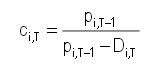# Price correction factor for BRD and SIF2 shares in BVB indices starting from May 9th

5/8/2019

Bucharest Stock Exchange (BVB) announces that, starting from the 9th of May 2019:

• the share price correction factor ci,T for 2018 dividends of BRD GROUPE SOCIETE GENERALE S.A. (BRD) that will be considered in calculating BET-TR and BET-XT-TR indexes is 1.138280.
• the share price correction factor ci,T for 2018 dividends of SIF MOLDOVA S.A. (SIF2) that will be considered in calculating the BET-XT-TR index is 1.026329.

The values of ci,T for BRD and SIF2 were determined according to the 4.2.4 article of BVB index management rules:

“4.2.4 Distribution of cash dividends

If an index constituent decides to distribute cash dividends to its shareholders, regardless of their source, the price correction factor ci,T is determined as follows:where

Pi,T-1   : closing price of symbol i at time T-1;

Di,T    : gross dividend payable for symbol i, for which time T is the ex-date”